# Chapter 28 Game Theory Game Theory n Game

• Slides: 92Chapter 28 Game Theory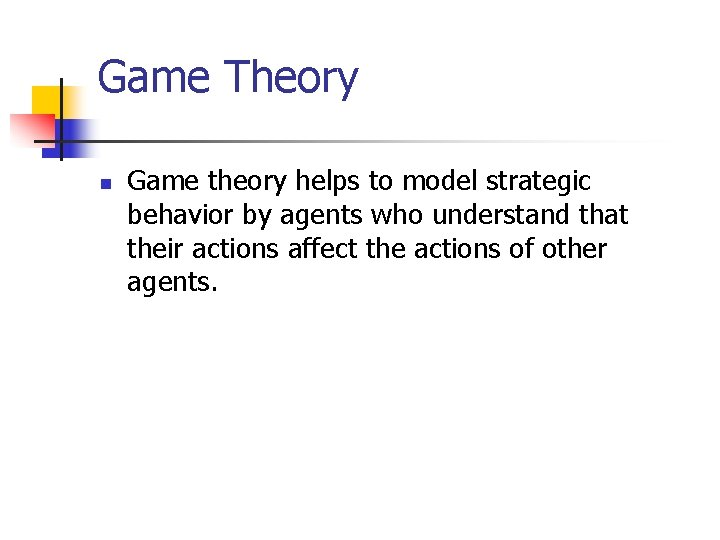Game Theory n Game theory helps to model strategic behavior by agents who understand that their actions affect the actions of other agents.Some Applications of Game Theory n n n The study of oligopolies (industries containing only a few firms) The study of cartels; e. g. OPEC The study of externalities; e. g. using a common resource such as a fishery. The study of military strategies. Bargaining. How markets work.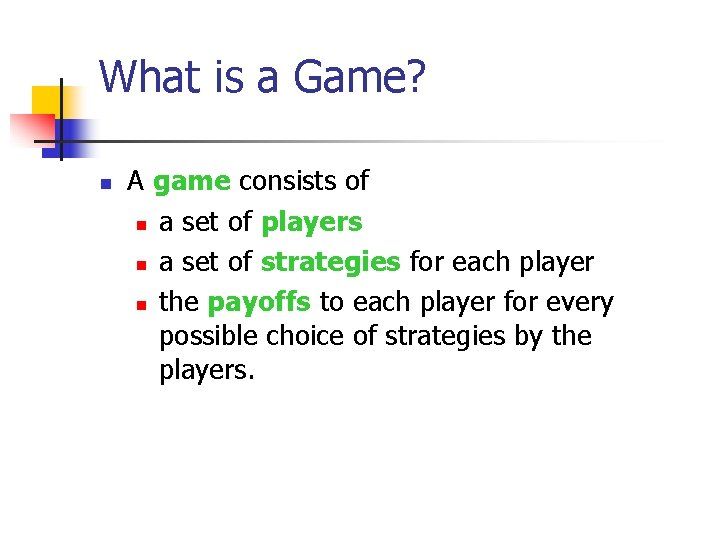What is a Game? n A game consists of n a set of players n a set of strategies for each player n the payoffs to each player for every possible choice of strategies by the players.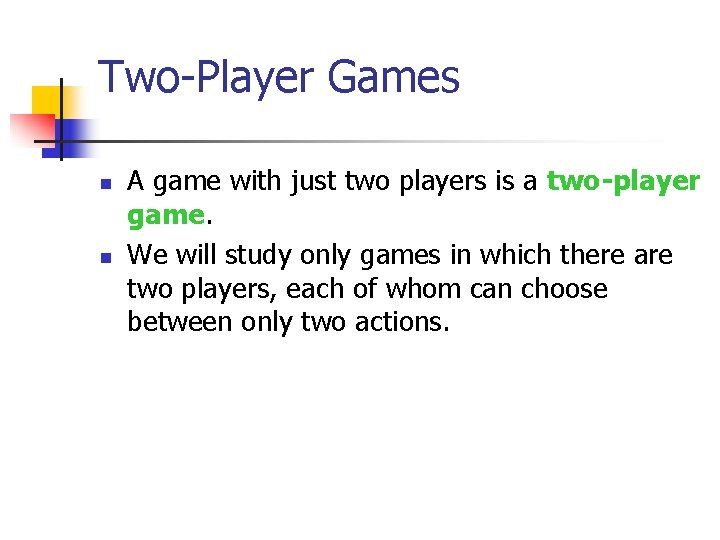Two-Player Games n n A game with just two players is a two-player game. We will study only games in which there are two players, each of whom can choose between only two actions.An Example of a Two-Player Game n n The players are called A and B. Player A has two actions, called “Up” and “Down”. Player B has two actions, called “Left” and “Right”. The table showing the payoffs to both players for each of the four possible action combinations is the game’s payoff matrix.An Example of a Two-Player Game Player B L Player A R U (3, 9) (1, 8) D (0, 0) (2, 1) This is the game’s payoff matrix. Player A’s payoff is shown first. Player B’s payoff is shown second.An Example of a Two-Player Game Player B L Player A R U (3, 9) (1, 8) D (0, 0) (2, 1) A play of the game is a pair such as (U, R) where the 1 st element is the action chosen by Player A and the 2 nd is the action chosen by Player B.An Example of a Two-Player Game Player B L Player A R U (3, 9) (1, 8) D (0, 0) (2, 1) This is the game’s payoff matrix. E. g. if A plays Up and B plays Right then A’s payoff is 1 and B’s payoff is 8.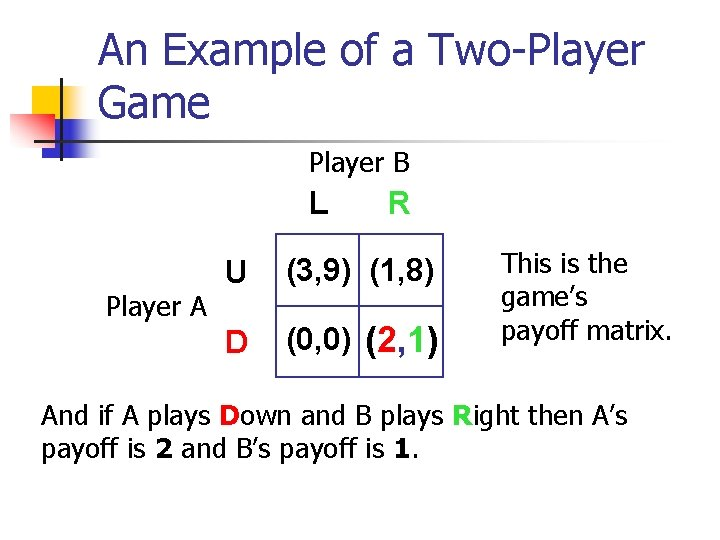An Example of a Two-Player Game Player B L Player A R U (3, 9) (1, 8) D (0, 0) (2, 1) This is the game’s payoff matrix. And if A plays Down and B plays Right then A’s payoff is 2 and B’s payoff is 1.An Example of a Two-Player Game Player B L Player A R U (3, 9) (1, 8) D (0, 0) (2, 1) What plays are we likely to see for this game?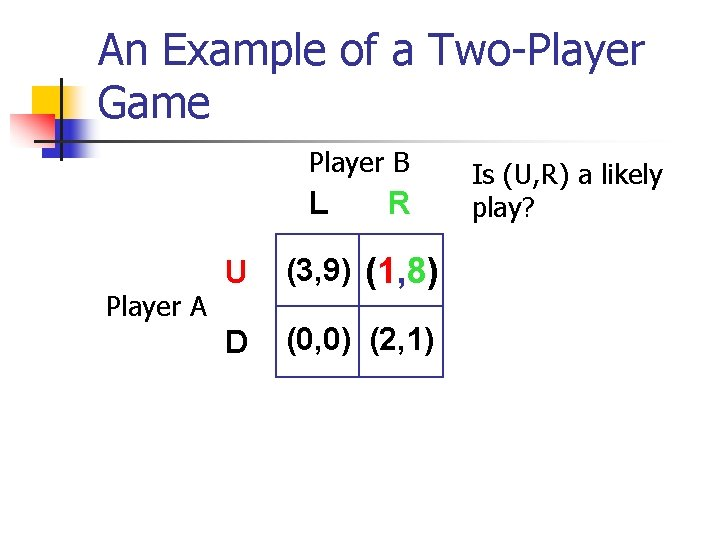An Example of a Two-Player Game Player B L Player A R U (3, 9) (1, 8) D (0, 0) (2, 1) Is (U, R) a likely play?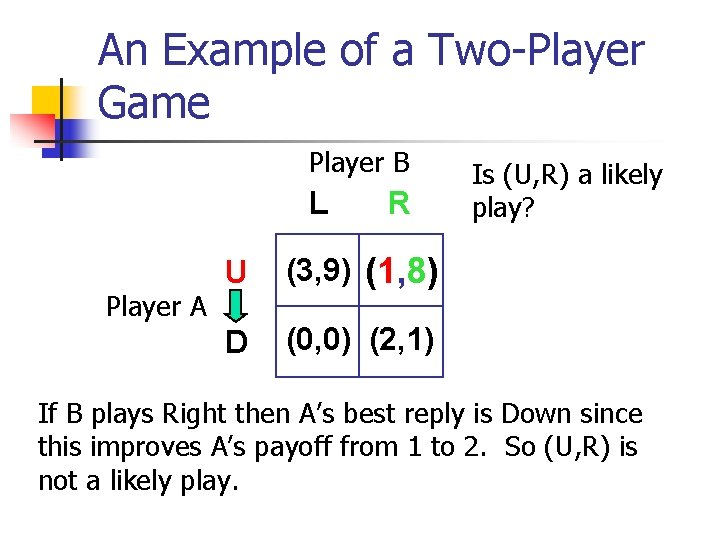An Example of a Two-Player Game Player B L Player A R U (3, 9) (1, 8) D (0, 0) (2, 1) Is (U, R) a likely play? If B plays Right then A’s best reply is Down since this improves A’s payoff from 1 to 2. So (U, R) is not a likely play.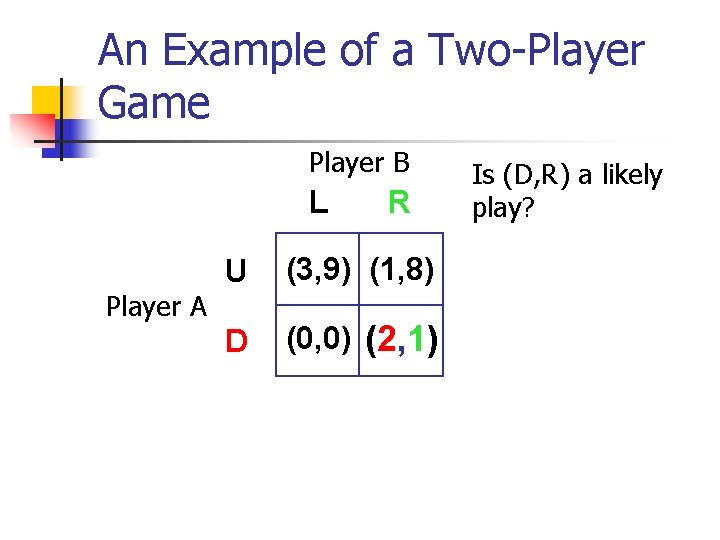An Example of a Two-Player Game Player B L Player A R U (3, 9) (1, 8) D (0, 0) (2, 1) Is (D, R) a likely play?An Example of a Two-Player Game Player B L Player A R U (3, 9) (1, 8) D (0, 0) (2, 1) Is (D, R) a likely play? If B plays Right then A’s best reply is Down.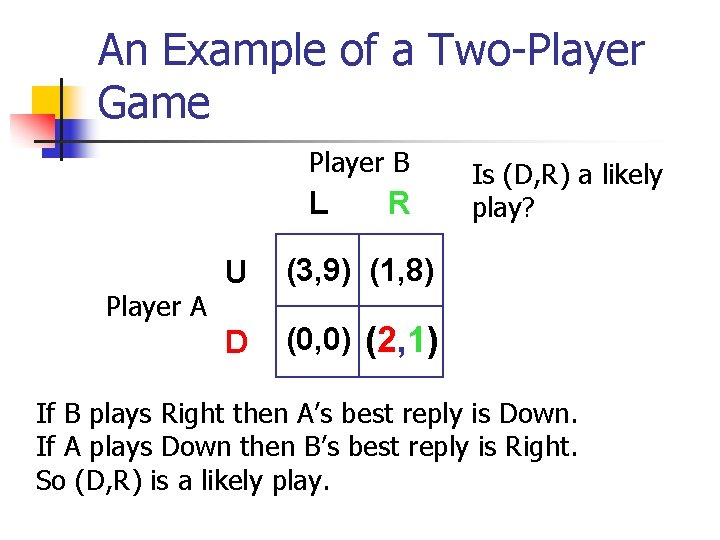An Example of a Two-Player Game Player B L Player A R U (3, 9) (1, 8) D (0, 0) (2, 1) Is (D, R) a likely play? If B plays Right then A’s best reply is Down. If A plays Down then B’s best reply is Right. So (D, R) is a likely play.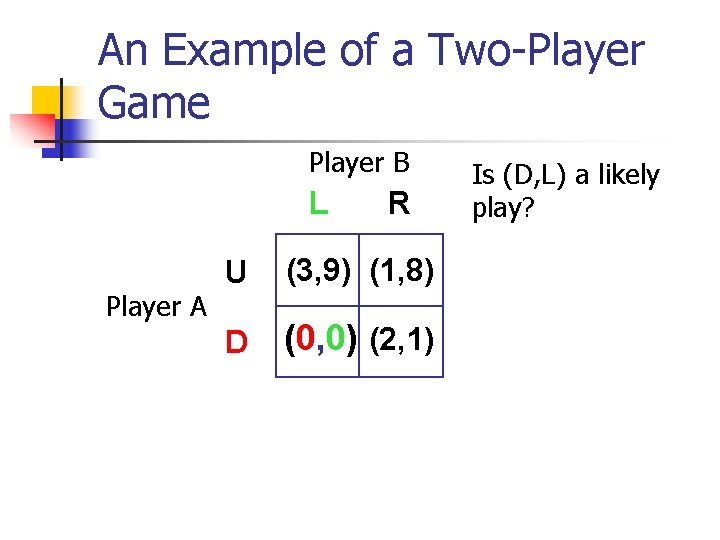An Example of a Two-Player Game Player B L Player A R U (3, 9) (1, 8) D (0, 0) (2, 1) Is (D, L) a likely play?An Example of a Two-Player Game Player B L Player A R U (3, 9) (1, 8) D (0, 0) (2, 1) Is (D, L) a likely play? If A plays Down then B’s best reply is Right, so (D, L) is not a likely play.An Example of a Two-Player Game Player B L Player A R U (3, 9) (1, 8) D (0, 0) (2, 1) Is (U, L) a likely play?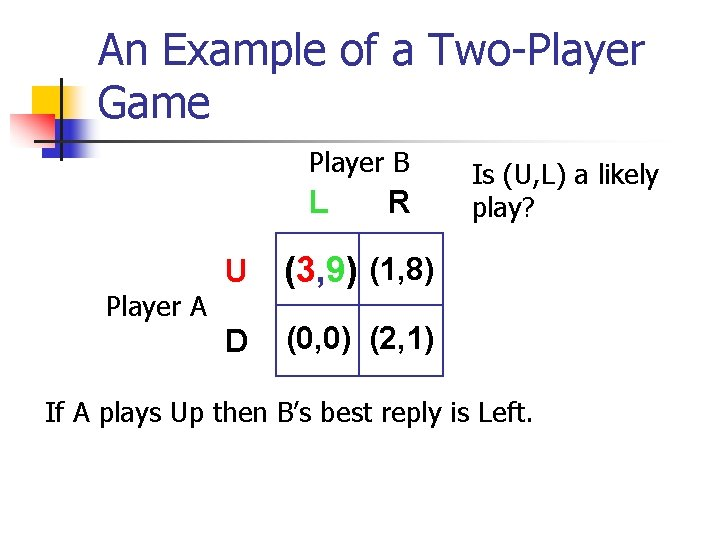An Example of a Two-Player Game Player B L Player A R U (3, 9) (1, 8) D (0, 0) (2, 1) Is (U, L) a likely play? If A plays Up then B’s best reply is Left.An Example of a Two-Player Game Player B L Player A R U (3, 9) (1, 8) D (0, 0) (2, 1) Is (U, L) a likely play? If A plays Up then B’s best reply is Left. If B plays Left then A’s best reply is Up. So (U, L) is a likely play.Nash Equilibrium n n A play of the game where each strategy is a best reply to the other is a Nash equilibrium. Our example has two Nash equilibria; (U, L) and (D, R).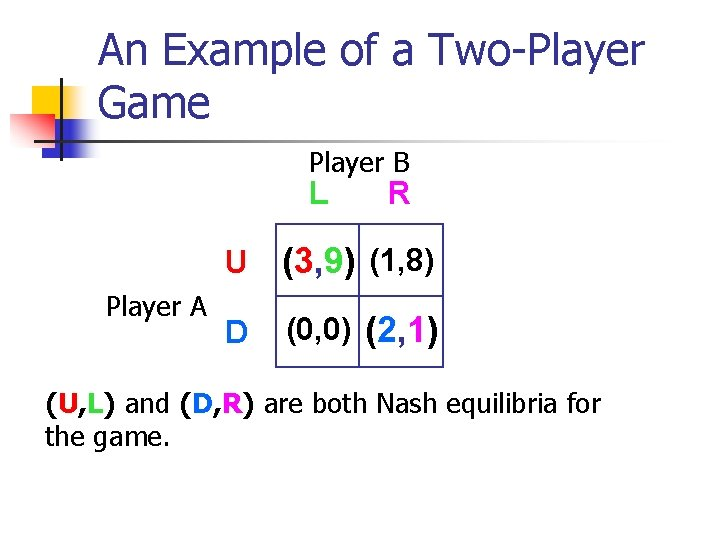An Example of a Two-Player Game Player B L Player A R U (3, 9) (1, 8) D (0, 0) (2, 1) (U, L) and (D, R) are both Nash equilibria for the game.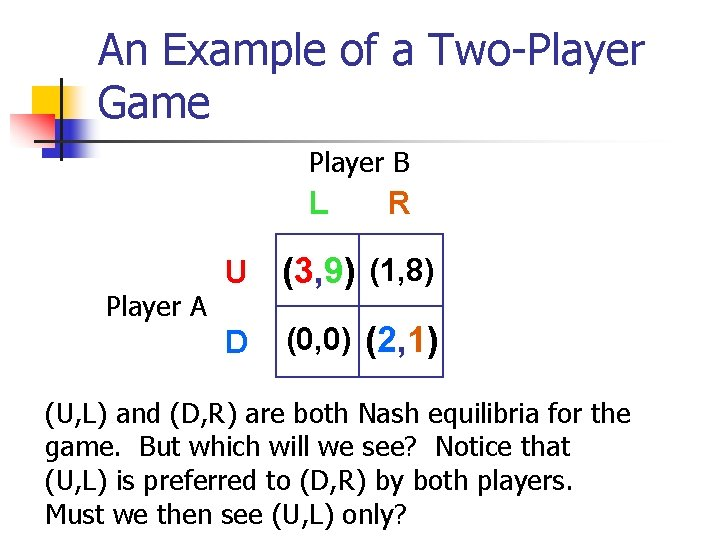An Example of a Two-Player Game Player B L Player A R U (3, 9) (1, 8) D (0, 0) (2, 1) (U, L) and (D, R) are both Nash equilibria for the game. But which will we see? Notice that (U, L) is preferred to (D, R) by both players. Must we then see (U, L) only?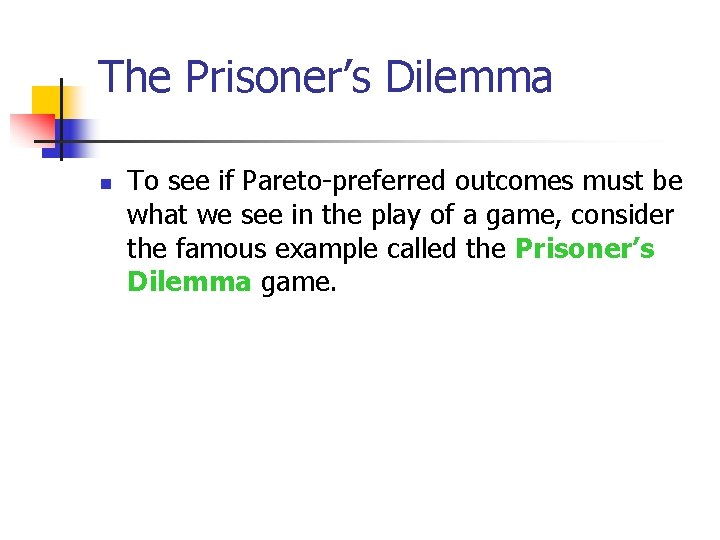The Prisoner’s Dilemma n To see if Pareto-preferred outcomes must be what we see in the play of a game, consider the famous example called the Prisoner’s Dilemma game.The Prisoner’s Dilemma Clyde Bonnie S S C (-5, -5) (-30, -1) C (-1, -30) (-10, -10) What plays are we likely to see for this game?The Prisoner’s Dilemma Clyde Bonnie S S C (-5, -5) (-30, -1) C (-1, -30) (-10, -10) If Bonnie plays Silence then Clyde’s best reply is Confess.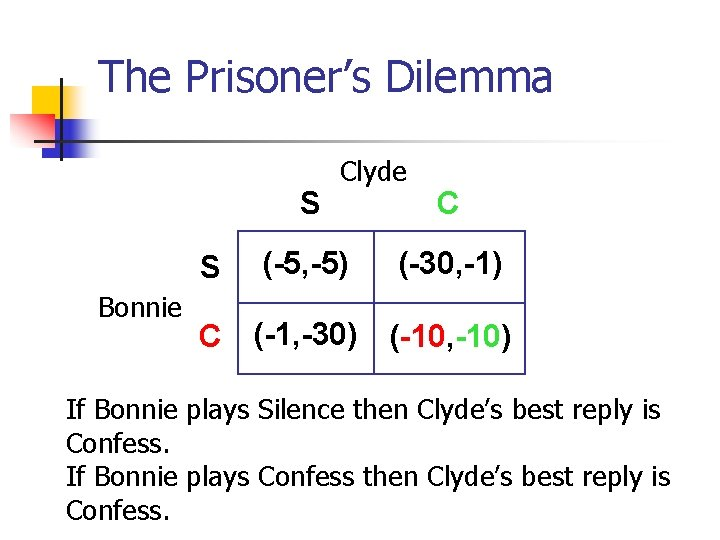The Prisoner’s Dilemma S S Bonnie Clyde (-5, -5) C (-30, -1) C (-1, -30) (-10, -10) If Bonnie plays Silence then Clyde’s best reply is Confess. If Bonnie plays Confess then Clyde’s best reply is Confess.The Prisoner’s Dilemma Clyde Bonnie S S C (-5, -5) (-30, -1) C (-1, -30) (-10, -10) So no matter what Bonnie plays, Clyde’s best reply is always Confess is a dominant strategy for Clyde.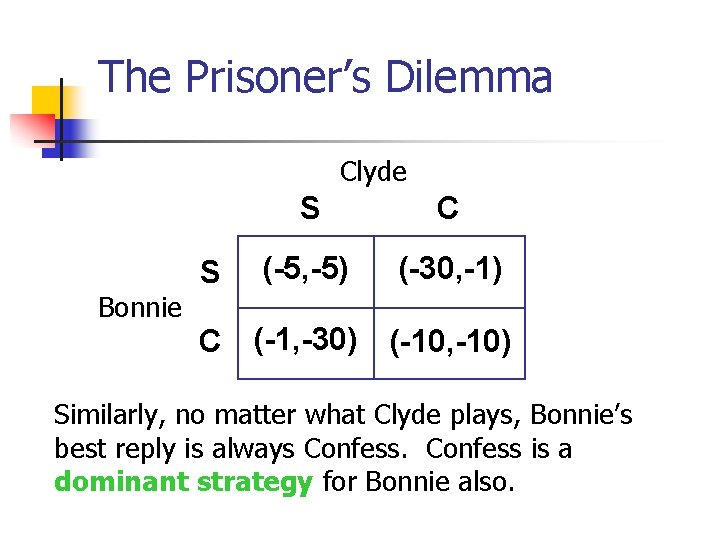The Prisoner’s Dilemma Clyde Bonnie S S C (-5, -5) (-30, -1) C (-1, -30) (-10, -10) Similarly, no matter what Clyde plays, Bonnie’s best reply is always Confess is a dominant strategy for Bonnie also.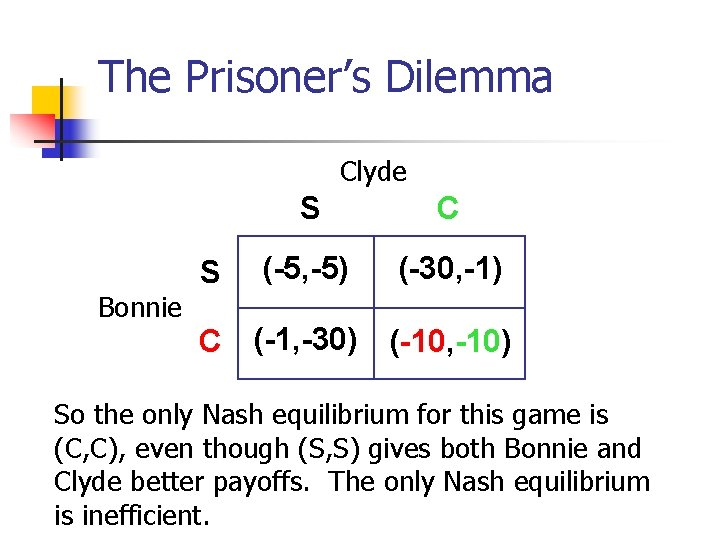The Prisoner’s Dilemma Clyde Bonnie S S C (-5, -5) (-30, -1) C (-1, -30) (-10, -10) So the only Nash equilibrium for this game is (C, C), even though (S, S) gives both Bonnie and Clyde better payoffs. The only Nash equilibrium is inefficient.Who Plays When? n n In both examples the players chose their strategies simultaneously. Such games are simultaneous play games.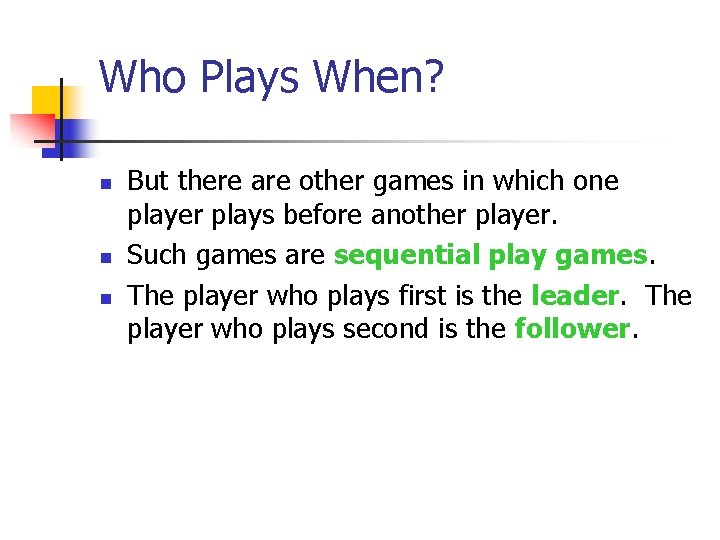Who Plays When? n n n But there are other games in which one player plays before another player. Such games are sequential play games. The player who plays first is the leader. The player who plays second is the follower.A Sequential Game Example n n Sometimes a game has more than one Nash equilibrium and it is hard to say which is more likely to occur. When a game is sequential it is sometimes possible to argue that one of the Nash equilibria is more likely to occur than the other.A Sequential Game Example Player B L Player A R U (3, 9) (1, 8) D (0, 0) (2, 1) (U, L) and (D, R) are both NE when this game is played simultaneously and we have no way of deciding which equilibrium is more likely to occur.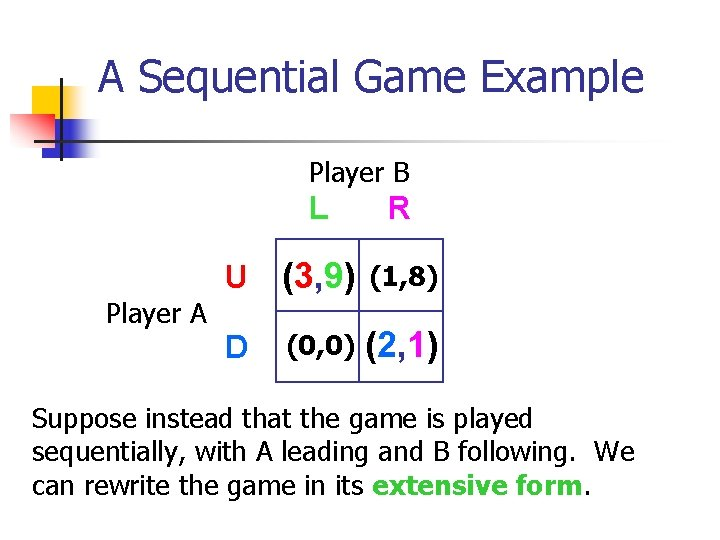A Sequential Game Example Player B Player A L R U (3, 9) (1, 8) D (0, 0) (2, 1) Suppose instead that the game is played sequentially, with A leading and B following. We can rewrite the game in its extensive form.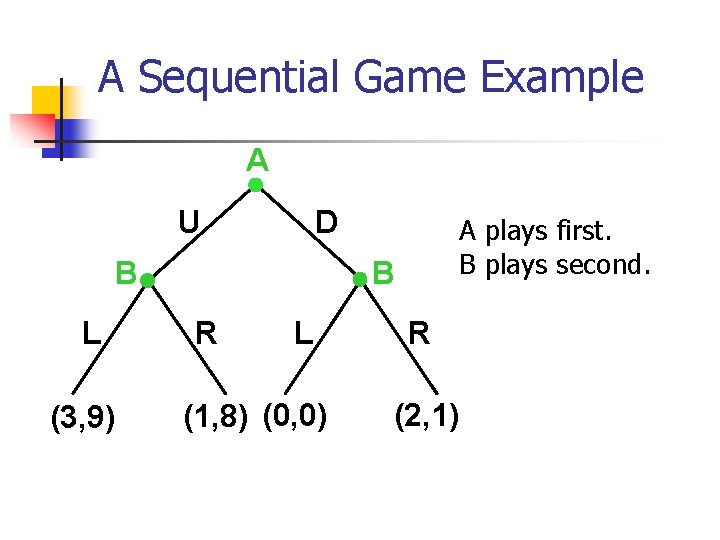A Sequential Game Example A U D B L (3, 9) A plays first. B plays second. B R L (1, 8) (0, 0) R (2, 1)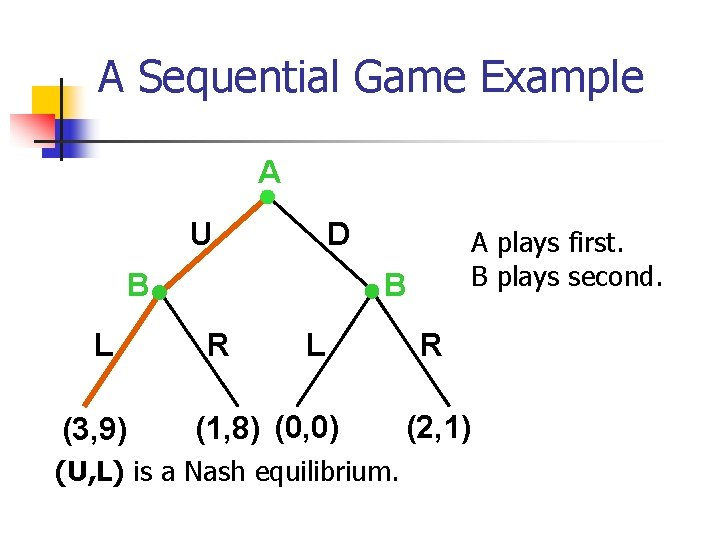A Sequential Game Example A U D B L (3, 9) A plays first. B plays second. B R L (1, 8) (0, 0) (U, L) is a Nash equilibrium. R (2, 1)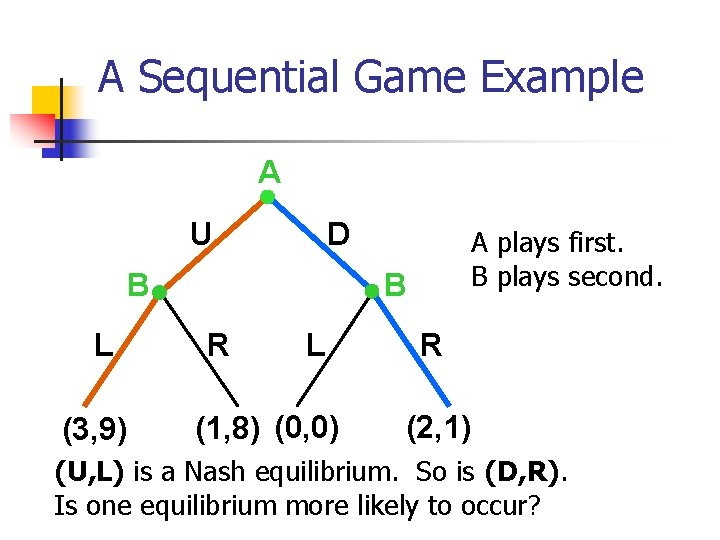A Sequential Game Example A U D B L (3, 9) A plays first. B plays second. B R L (1, 8) (0, 0) R (2, 1) (U, L) is a Nash equilibrium. So is (D, R). Is one equilibrium more likely to occur?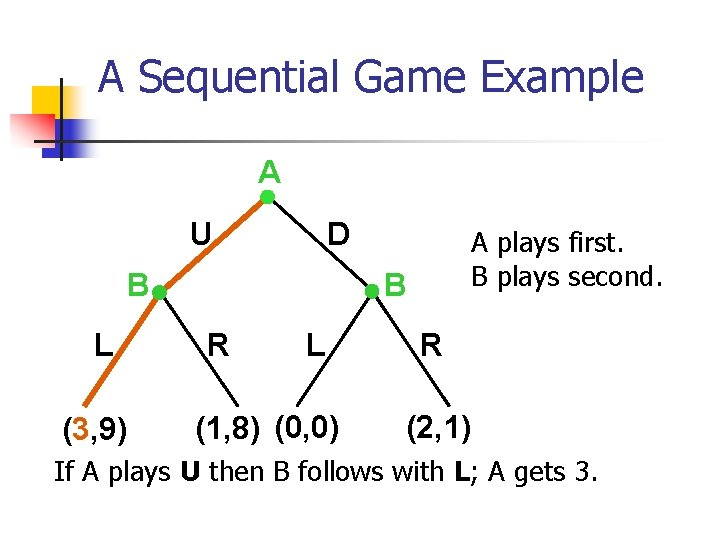A Sequential Game Example A U D B L (3, 9) A plays first. B plays second. B R L (1, 8) (0, 0) R (2, 1) If A plays U then B follows with L; A gets 3.A Sequential Game Example A U D B L (3, 9) A plays first. B plays second. B R L (1, 8) (0, 0) R (2, 1) If A plays U then B follows with L; A gets 3. If A plays D then B follows with R; A gets 2.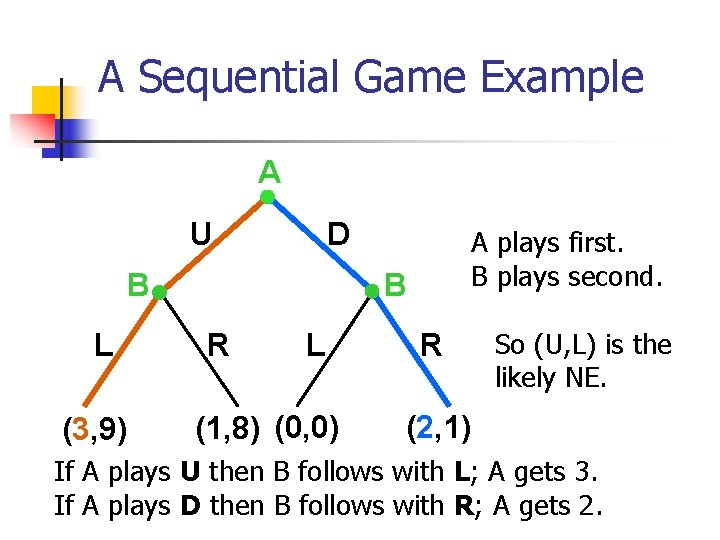A Sequential Game Example A U D B L (3, 9) A plays first. B plays second. B R L (1, 8) (0, 0) R So (U, L) is the likely NE. (2, 1) If A plays U then B follows with L; A gets 3. If A plays D then B follows with R; A gets 2.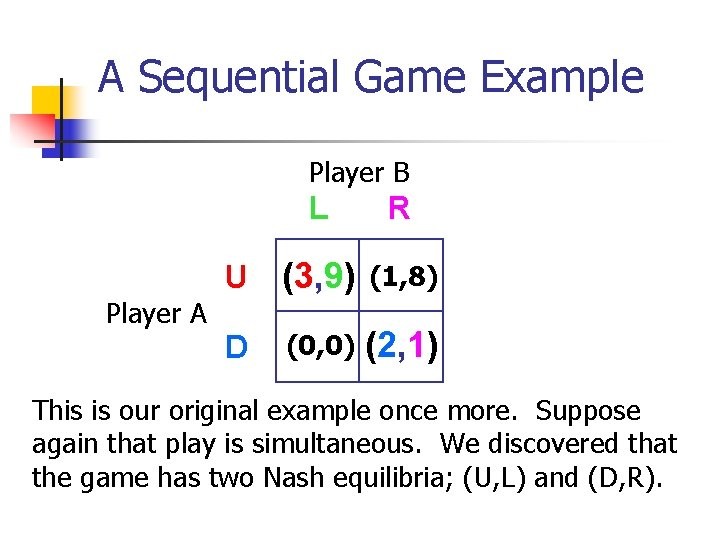A Sequential Game Example Player B Player A L R U (3, 9) (1, 8) D (0, 0) (2, 1) This is our original example once more. Suppose again that play is simultaneous. We discovered that the game has two Nash equilibria; (U, L) and (D, R).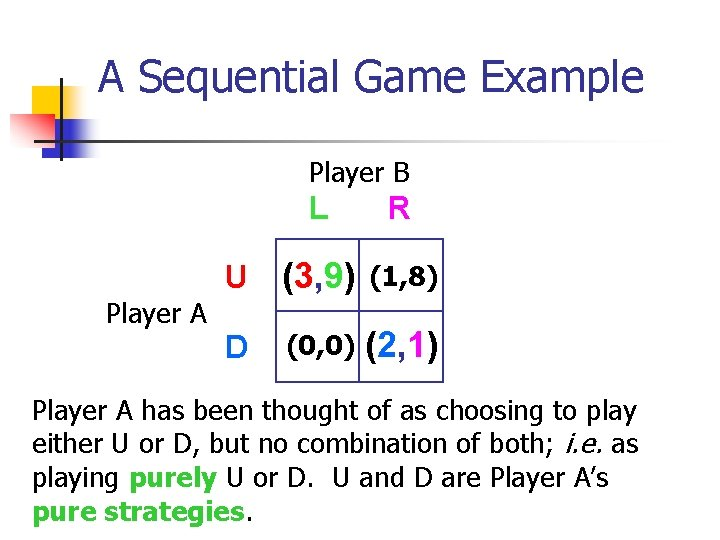A Sequential Game Example Player B Player A L R U (3, 9) (1, 8) D (0, 0) (2, 1) Player A has been thought of as choosing to play either U or D, but no combination of both; i. e. as playing purely U or D. U and D are Player A’s pure strategies.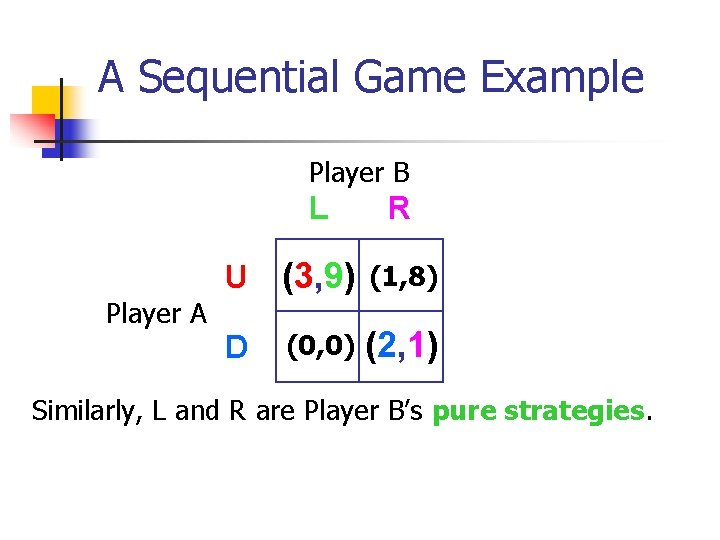A Sequential Game Example Player B Player A L R U (3, 9) (1, 8) D (0, 0) (2, 1) Similarly, L and R are Player B’s pure strategies.A Sequential Game Example Player B Player A L R U (3, 9) (1, 8) D (0, 0) (2, 1) Consequently, (U, L) and (D, R) are pure strategy Nash equilibria. Must every game have at least one pure strategy Nash equilibrium?Pure Strategies Player B Player A L R U (1, 2) (0, 4) D (0, 5) (3, 2) Here is a new game. Are there any pure strategy Nash equilibria?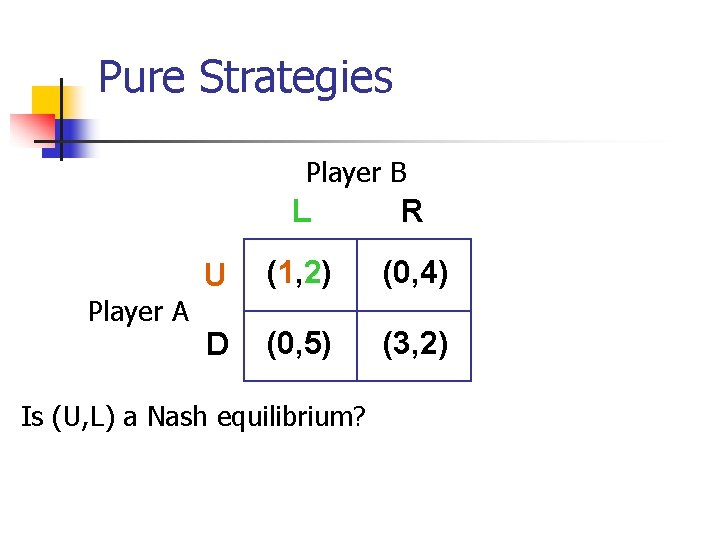Pure Strategies Player B Player A L R U (1, 2) (0, 4) D (0, 5) (3, 2) Is (U, L) a Nash equilibrium?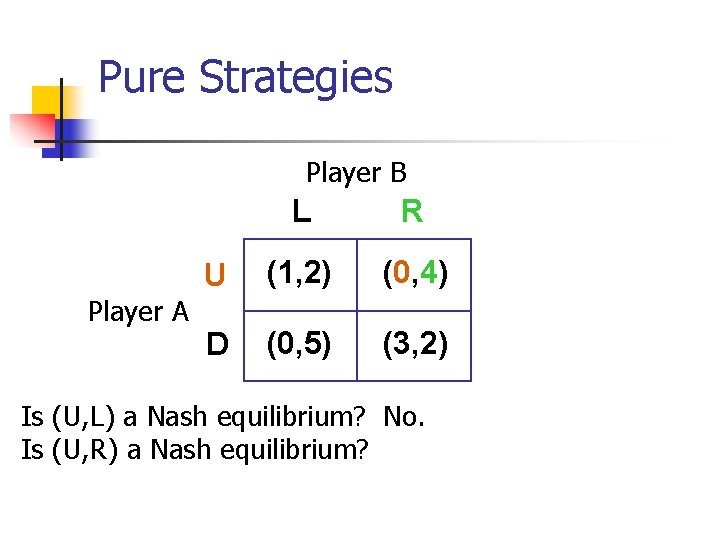Pure Strategies Player B Player A L R U (1, 2) (0, 4) D (0, 5) (3, 2) Is (U, L) a Nash equilibrium? No. Is (U, R) a Nash equilibrium?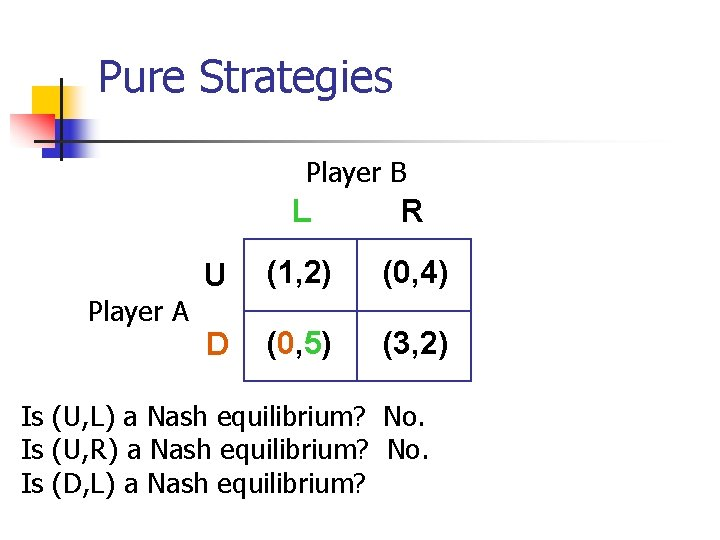Pure Strategies Player B Player A L R U (1, 2) (0, 4) D (0, 5) (3, 2) Is (U, L) a Nash equilibrium? No. Is (U, R) a Nash equilibrium? No. Is (D, L) a Nash equilibrium?Pure Strategies Player B Player A Is Is L R U (1, 2) (0, 4) D (0, 5) (3, 2) (U, L) a Nash equilibrium? No. (U, R) a Nash equilibrium? No. (D, L) a Nash equilibrium? No. (D, R) a Nash equilibrium?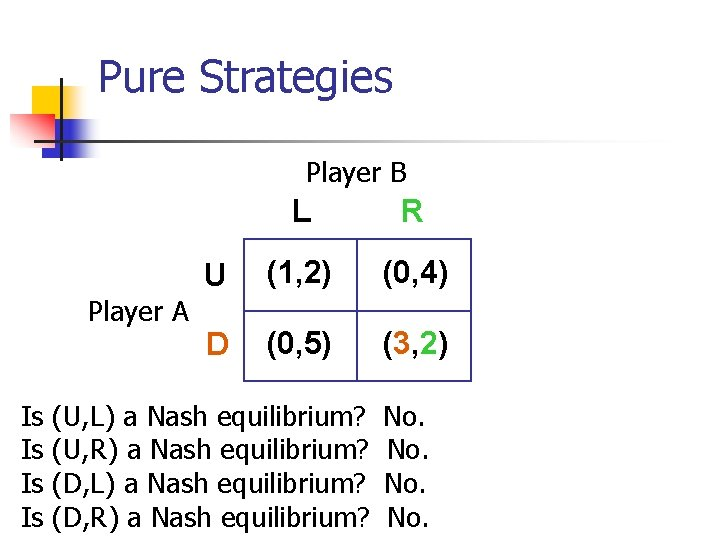Pure Strategies Player B Player A Is Is L R U (1, 2) (0, 4) D (0, 5) (3, 2) (U, L) a Nash equilibrium? (U, R) a Nash equilibrium? (D, L) a Nash equilibrium? (D, R) a Nash equilibrium? No. No.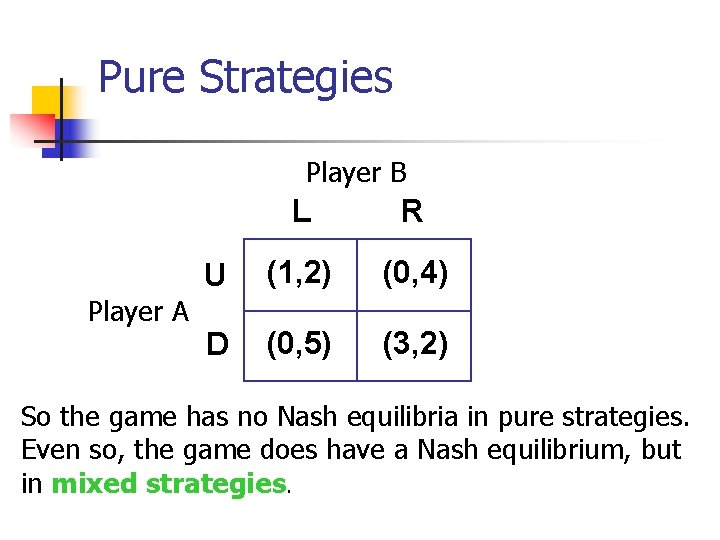Pure Strategies Player B Player A L R U (1, 2) (0, 4) D (0, 5) (3, 2) So the game has no Nash equilibria in pure strategies. Even so, the game does have a Nash equilibrium, but in mixed strategies.Mixed Strategies n n n Instead of playing purely Up or Down, Player A selects a probability distribution ( U, 1 - U), meaning that with probability U Player A will play Up and with probability 1 - U will play Down. Player A is mixing over the pure strategies Up and Down. The probability distribution (p. U, 1 -p. U) is a mixed strategy for Player A.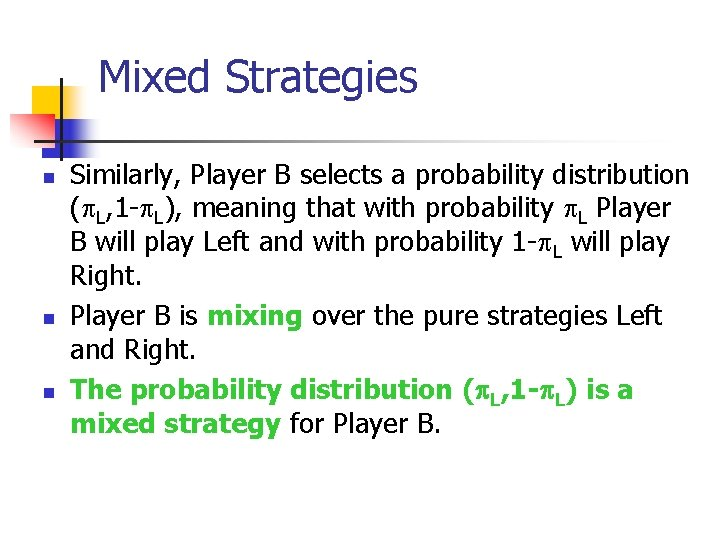Mixed Strategies n n n Similarly, Player B selects a probability distribution ( L, 1 - L), meaning that with probability L Player B will play Left and with probability 1 - L will play Right. Player B is mixing over the pure strategies Left and Right. The probability distribution (p. L, 1 -p. L) is a mixed strategy for Player B.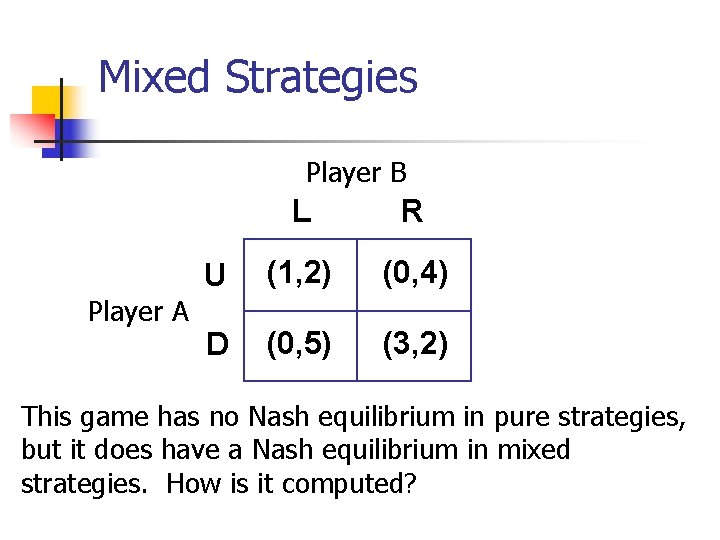Mixed Strategies Player B Player A L R U (1, 2) (0, 4) D (0, 5) (3, 2) This game has no Nash equilibrium in pure strategies, but it does have a Nash equilibrium in mixed strategies. How is it computed?Mixed Strategies Player B L, L R, 1 - L U, U Player A D, 1 - U (1, 2) (0, 4) (0, 5) (3, 2)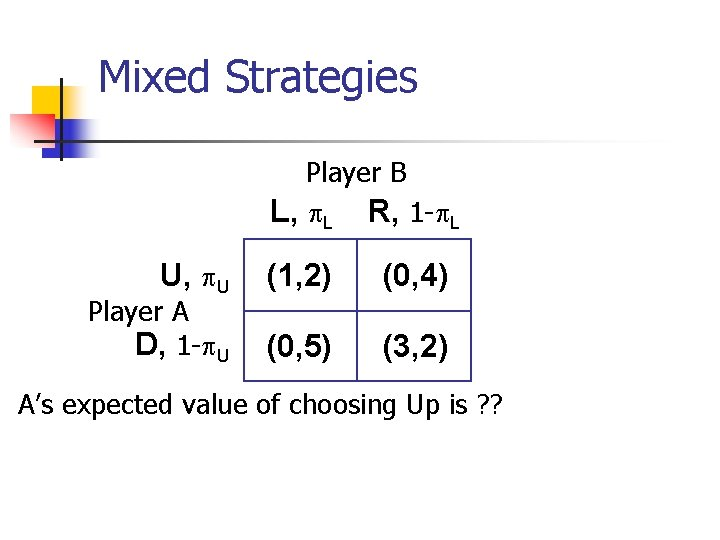Mixed Strategies Player B L, L R, 1 - L U, U Player A D, 1 - U (1, 2) (0, 4) (0, 5) (3, 2) A’s expected value of choosing Up is ? ?Mixed Strategies Player B L, L R, 1 - L U, U Player A D, 1 - U (1, 2) (0, 4) (0, 5) (3, 2) A’s expected value of choosing Up is L. A’s expected value of choosing Down is ? ?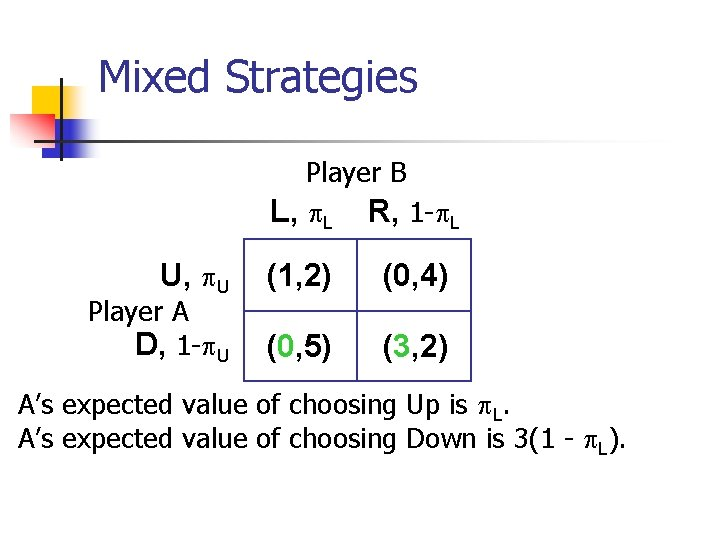Mixed Strategies Player B L, L R, 1 - L U, U Player A D, 1 - U (1, 2) (0, 4) (0, 5) (3, 2) A’s expected value of choosing Up is L. A’s expected value of choosing Down is 3(1 - L).Mixed Strategies Player B L, L R, 1 - L U, U Player A D, 1 - U (1, 2) (0, 4) (0, 5) (3, 2) A’s expected value of choosing Up is L. A’s expected value of choosing Down is 3(1 - L). If L > 3(1 - L) then A will choose only Up, but there is no NE in which A plays only Up.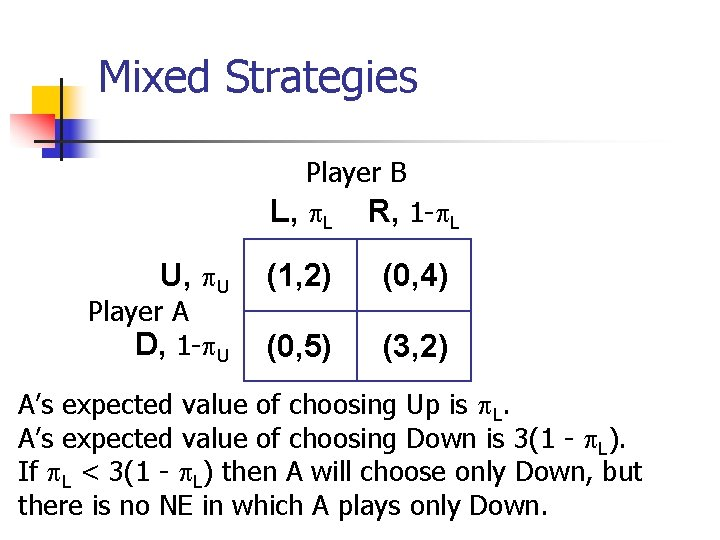Mixed Strategies Player B L, L R, 1 - L U, U Player A D, 1 - U (1, 2) (0, 4) (0, 5) (3, 2) A’s expected value of choosing Up is L. A’s expected value of choosing Down is 3(1 - L). If L < 3(1 - L) then A will choose only Down, but there is no NE in which A plays only Down.Mixed Strategies Player B L, L R, 1 - L U, U Player A D, 1 - U (1, 2) (0, 4) (0, 5) (3, 2) If there is a NE necessarily L = 3(1 - L) L = 3/4; i. e. the way B mixes over Left and Right must make A indifferent between choosing Up or Down.Mixed Strategies Player B L, 3/4 R, 1/4 U, U Player A D, 1 - U (1, 2) (0, 4) (0, 5) (3, 2) If there is a NE necessarily L = 3(1 - L) L = 3/4; i. e. the way B mixes over Left and Right must make A indifferent between choosing Up or Down.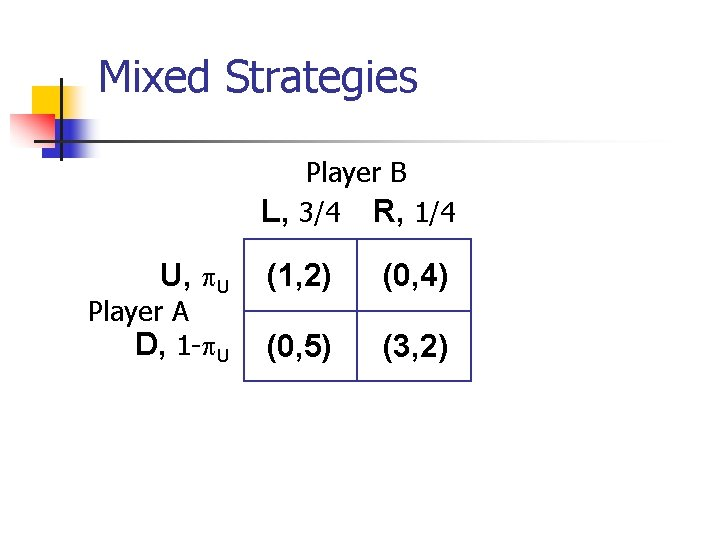Mixed Strategies Player B L, 3/4 R, 1/4 U, U Player A D, 1 - U (1, 2) (0, 4) (0, 5) (3, 2)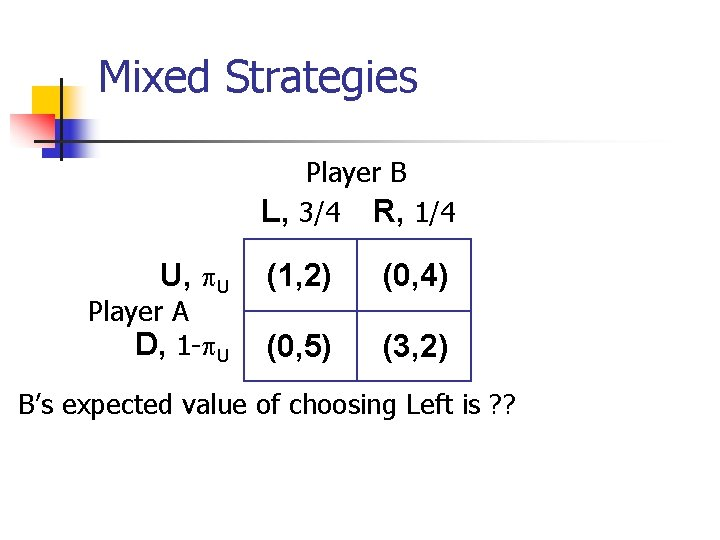Mixed Strategies Player B L, 3/4 R, 1/4 U, U Player A D, 1 - U (1, 2) (0, 4) (0, 5) (3, 2) B’s expected value of choosing Left is ? ?Mixed Strategies Player B L, 3/4 R, 1/4 U, U Player A D, 1 - U (1, 2) (0, 4) (0, 5) (3, 2) B’s expected value of choosing Left is 2 U + 5(1 - U). B’s expected value of choosing Right is ? ?Mixed Strategies Player B L, 3/4 R, 1/4 U, U Player A D, 1 - U (1, 2) (0, 4) (0, 5) (3, 2) B’s expected value of choosing Left is 2 U + 5(1 - U). B’s expected value of choosing Right is 4 U + 2(1 - U).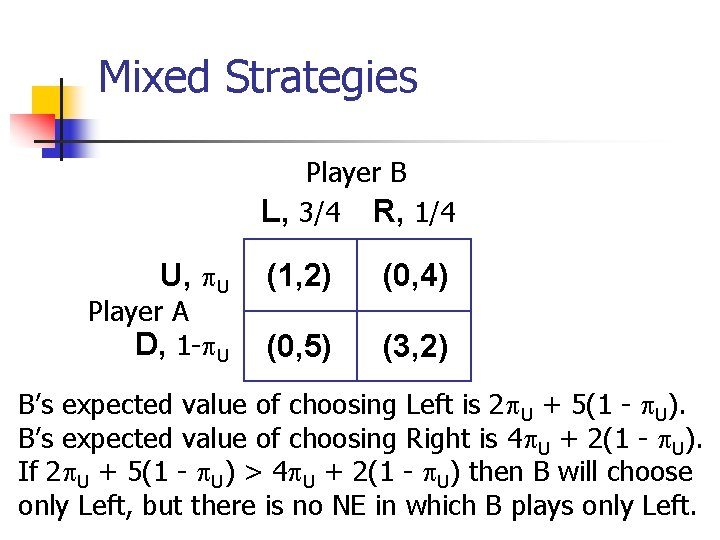Mixed Strategies Player B L, 3/4 R, 1/4 U, U Player A D, 1 - U (1, 2) (0, 4) (0, 5) (3, 2) B’s expected value of choosing Left is 2 U + 5(1 - U). B’s expected value of choosing Right is 4 U + 2(1 - U). If 2 U + 5(1 - U) > 4 U + 2(1 - U) then B will choose only Left, but there is no NE in which B plays only Left.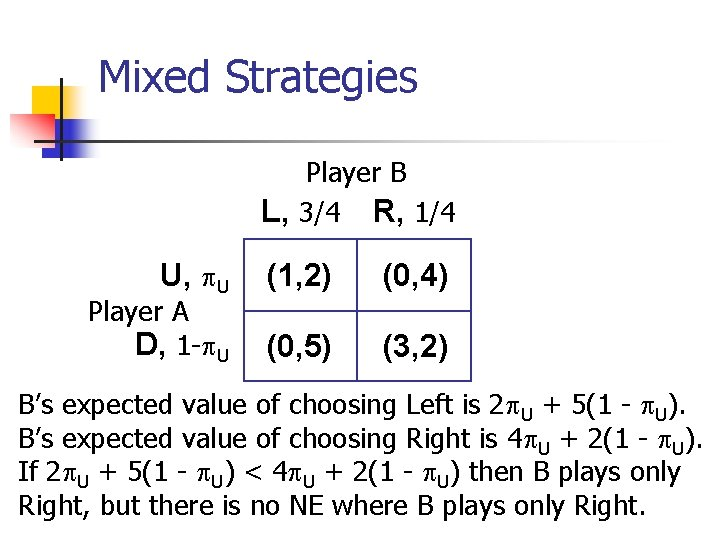Mixed Strategies Player B L, 3/4 R, 1/4 U, U Player A D, 1 - U (1, 2) (0, 4) (0, 5) (3, 2) B’s expected value of choosing Left is 2 U + 5(1 - U). B’s expected value of choosing Right is 4 U + 2(1 - U). If 2 U + 5(1 - U) < 4 U + 2(1 - U) then B plays only Right, but there is no NE where B plays only Right.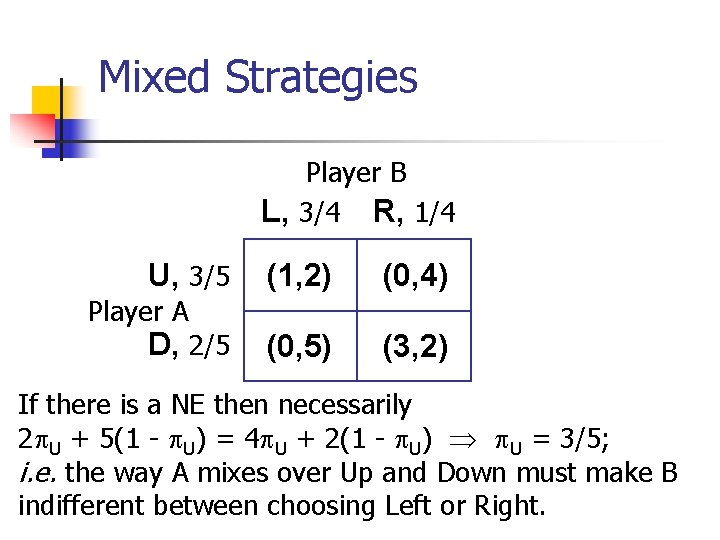Mixed Strategies Player B L, 3/4 R, 1/4 U, 3/5 (1, 2) (0, 4) (0, 5) (3, 2) Player A D, 2/5 If there is a NE then necessarily 2 U + 5(1 - U) = 4 U + 2(1 - U) U = 3/5; i. e. the way A mixes over Up and Down must make B indifferent between choosing Left or Right.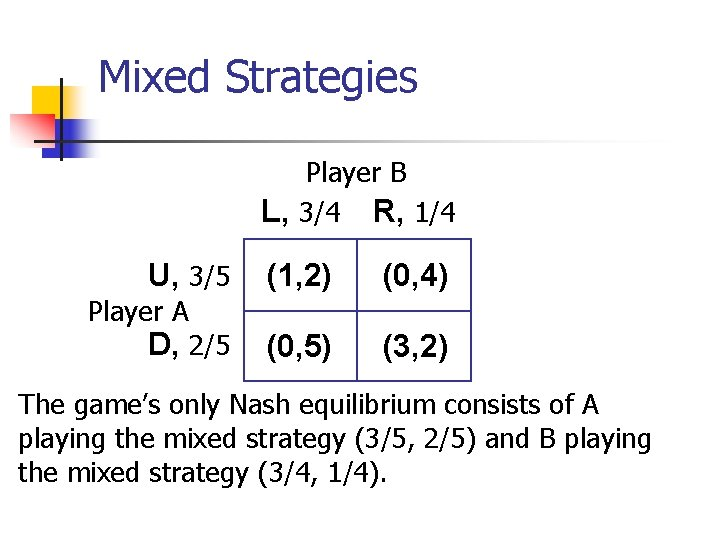Mixed Strategies Player B L, 3/4 R, 1/4 U, 3/5 (1, 2) (0, 4) (0, 5) (3, 2) Player A D, 2/5 The game’s only Nash equilibrium consists of A playing the mixed strategy (3/5, 2/5) and B playing the mixed strategy (3/4, 1/4).Mixed Strategies Player B L, 3/4 R, 1/4 U, 3/5 Player A D, 2/5 (1, 2) 9/20 (0, 5) (0, 4) (3, 2) The payoff will be (1, 2) with probability 3/5 × 3/4 = 9/20.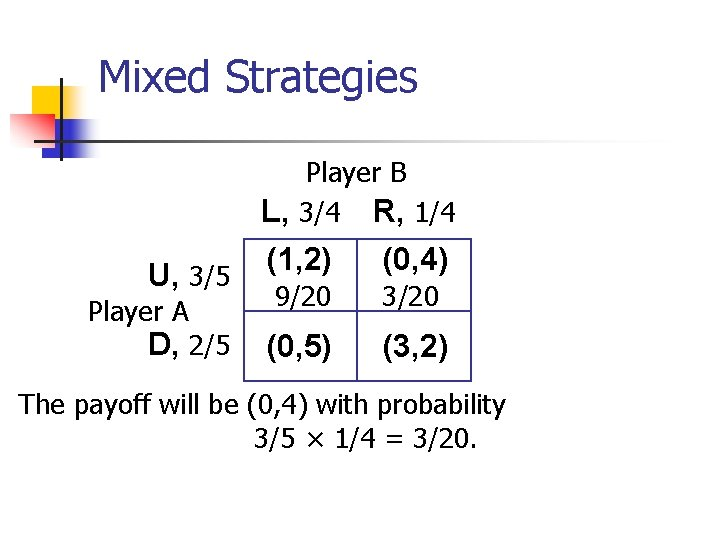Mixed Strategies Player B L, 3/4 R, 1/4 U, 3/5 Player A D, 2/5 (1, 2) (0, 4) 9/20 3/20 (0, 5) (3, 2) The payoff will be (0, 4) with probability 3/5 × 1/4 = 3/20.Mixed Strategies Player B L, 3/4 R, 1/4 U, 3/5 Player A D, 2/5 (1, 2) (0, 4) 9/20 3/20 (0, 5) (3, 2) 6/20 The payoff will be (0, 5) with probability 2/5 × 3/4 = 6/20.Mixed Strategies Player B L, 3/4 R, 1/4 U, 3/5 Player A D, 2/5 (1, 2) (0, 4) 9/20 3/20 (0, 5) (3, 2) 6/20 2/20 The payoff will be (3, 2) with probability 2/5 × 1/4 = 2/20.Mixed Strategies Player B L, 3/4 R, 1/4 U, 3/5 Player A D, 2/5 (1, 2) (0, 4) 9/20 3/20 (0, 5) (3, 2) 6/20 2/20 A’s NE expected payoff is 1× 9/20 + 3× 2/20 = 3/4.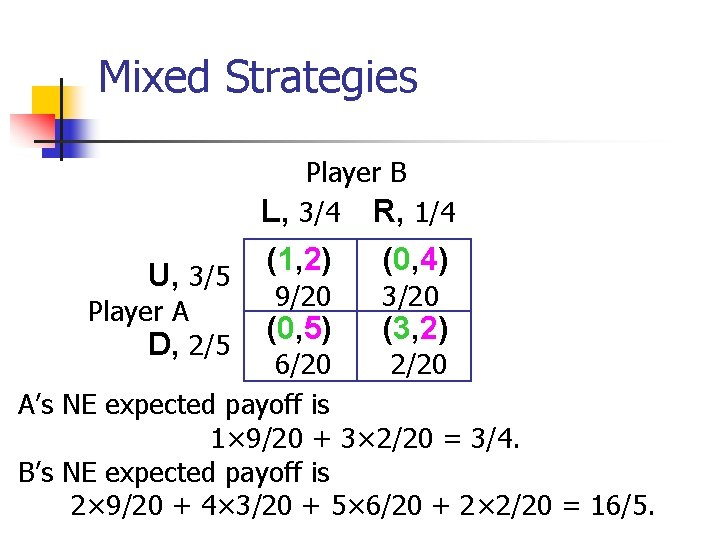Mixed Strategies Player B L, 3/4 R, 1/4 U, 3/5 Player A D, 2/5 (1, 2) (0, 4) 9/20 3/20 (0, 5) (3, 2) 6/20 2/20 A’s NE expected payoff is 1× 9/20 + 3× 2/20 = 3/4. B’s NE expected payoff is 2× 9/20 + 4× 3/20 + 5× 6/20 + 2× 2/20 = 16/5.How Many Nash Equilibria? n n A game with a finite number of players, each with a finite number of pure strategies, has at least one Nash equilibrium. So if the game has no pure strategy Nash equilibrium then it must have at least one mixed strategy Nash equilibrium.Repeated Games n n A strategic game that is repeated by being played once in each of a number of periods. What strategies are sensible for the players depends greatly on whether or not the game n is repeated over only a finite number of periods n is repeated over an infinite number of periods.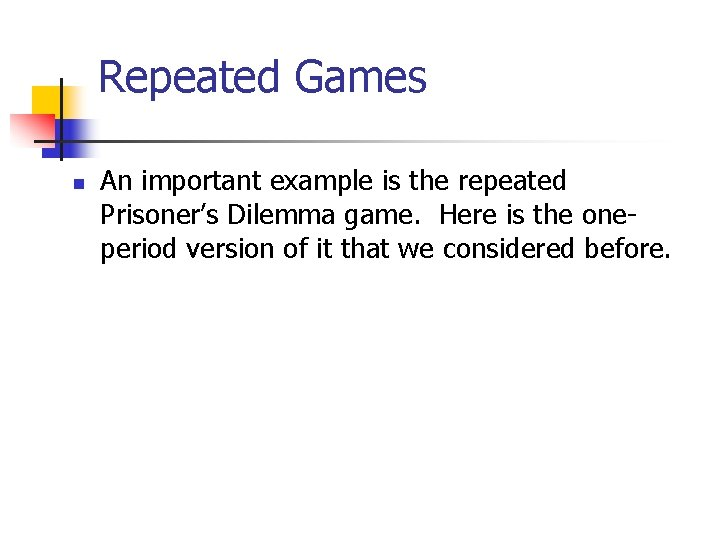Repeated Games n An important example is the repeated Prisoner’s Dilemma game. Here is the oneperiod version of it that we considered before.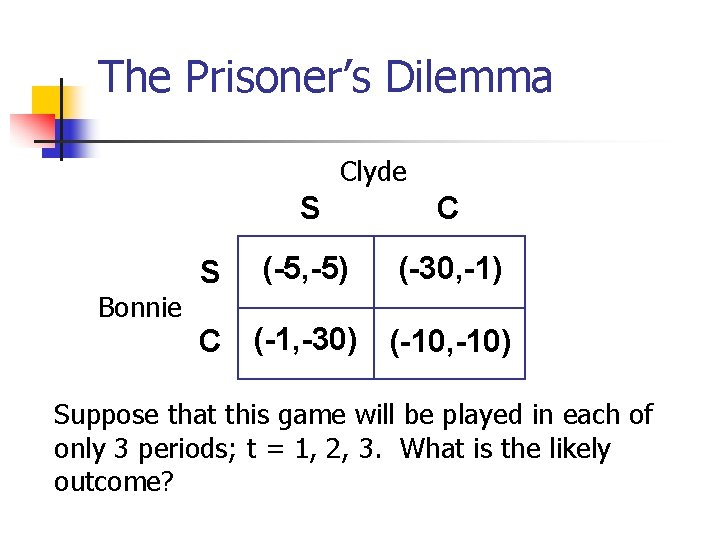The Prisoner’s Dilemma Clyde Bonnie S S C (-5, -5) (-30, -1) C (-1, -30) (-10, -10) Suppose that this game will be played in each of only 3 periods; t = 1, 2, 3. What is the likely outcome?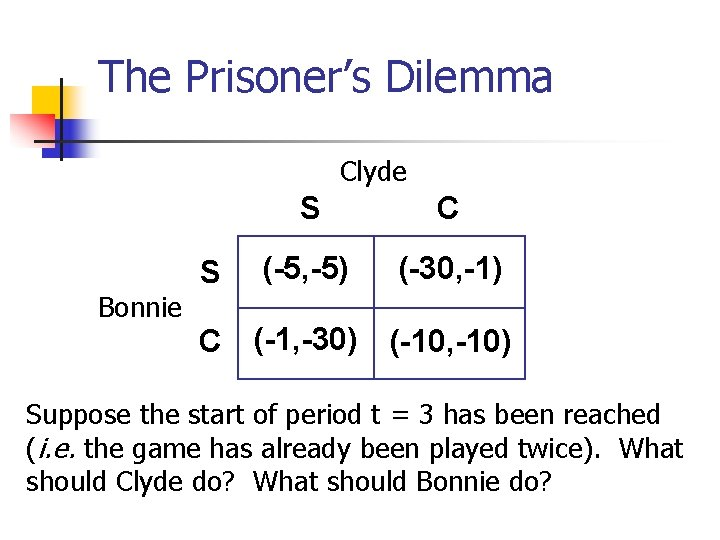The Prisoner’s Dilemma Clyde Bonnie S S C (-5, -5) (-30, -1) C (-1, -30) (-10, -10) Suppose the start of period t = 3 has been reached (i. e. the game has already been played twice). What should Clyde do? What should Bonnie do?The Prisoner’s Dilemma Clyde Bonnie S S C (-5, -5) (-30, -1) C (-1, -30) (-10, -10) Suppose the start of period t = 3 has been reached (i. e. the game has already been played twice). What should Clyde do? What should Bonnie do? Both should choose Confess.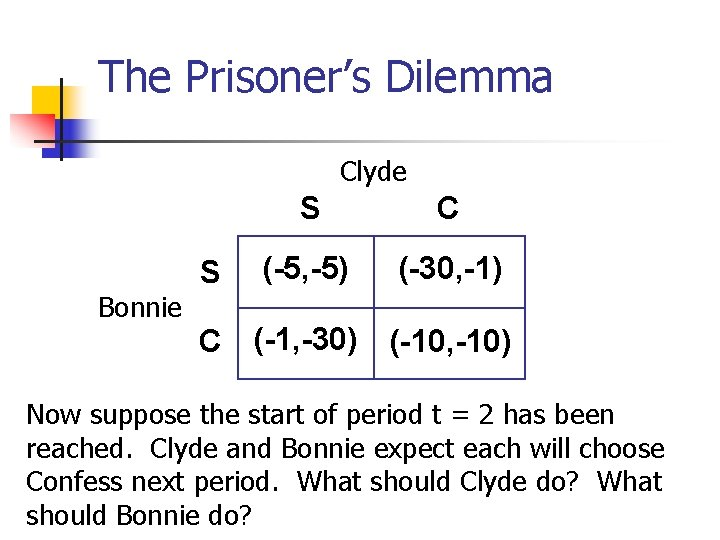The Prisoner’s Dilemma Clyde Bonnie S S C (-5, -5) (-30, -1) C (-1, -30) (-10, -10) Now suppose the start of period t = 2 has been reached. Clyde and Bonnie expect each will choose Confess next period. What should Clyde do? What should Bonnie do?The Prisoner’s Dilemma Clyde Bonnie S S C (-5, -5) (-30, -1) C (-1, -30) (-10, -10) Now suppose the start of period t = 2 has been reached. Clyde and Bonnie expect each will choose Confess next period. What should Clyde do? What should Bonnie do? Both should choose Confess.The Prisoner’s Dilemma Clyde Bonnie S S C (-5, -5) (-30, -1) C (-1, -30) (-10, -10) At the start of period t = 1 Clyde and Bonnie both expect that each will choose Confess in each of the next two periods. What should Clyde do? What should Bonnie do?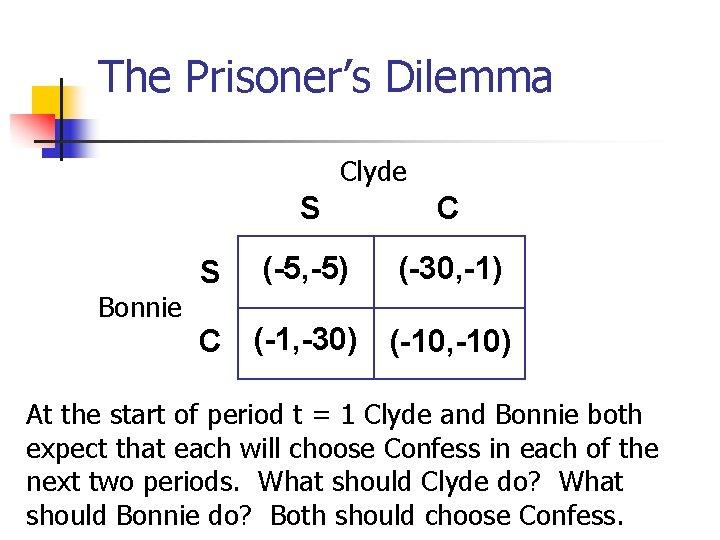The Prisoner’s Dilemma Clyde Bonnie S S C (-5, -5) (-30, -1) C (-1, -30) (-10, -10) At the start of period t = 1 Clyde and Bonnie both expect that each will choose Confess in each of the next two periods. What should Clyde do? What should Bonnie do? Both should choose Confess.The Prisoner’s Dilemma Clyde Bonnie S S C (-5, -5) (-30, -1) C (-1, -30) (-10, -10) The only credible (subgame perfect) NE for this game is where both Clyde and Bonnie choose Confess in every period.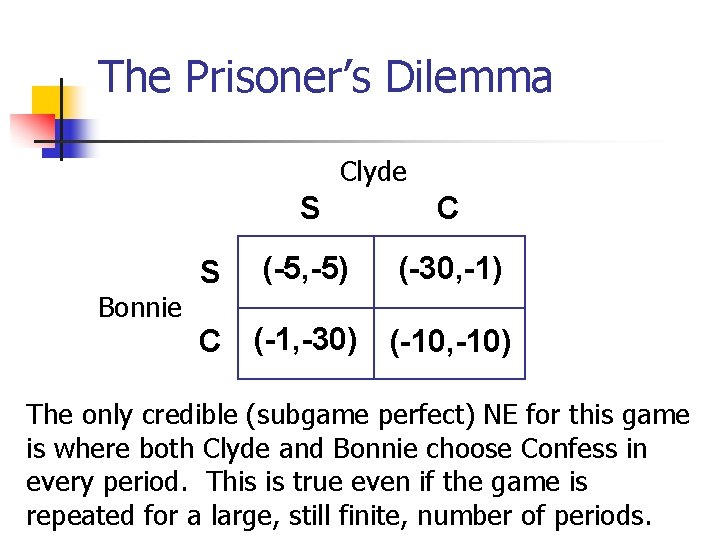The Prisoner’s Dilemma Clyde Bonnie S S C (-5, -5) (-30, -1) C (-1, -30) (-10, -10) The only credible (subgame perfect) NE for this game is where both Clyde and Bonnie choose Confess in every period. This is true even if the game is repeated for a large, still finite, number of periods.The Prisoner’s Dilemma Clyde Bonnie S S C (-5, -5) (-30, -1) C (-1, -30) (-10, -10) However, if the game is repeated for an infinite number of periods then the game has a huge number of credible NE.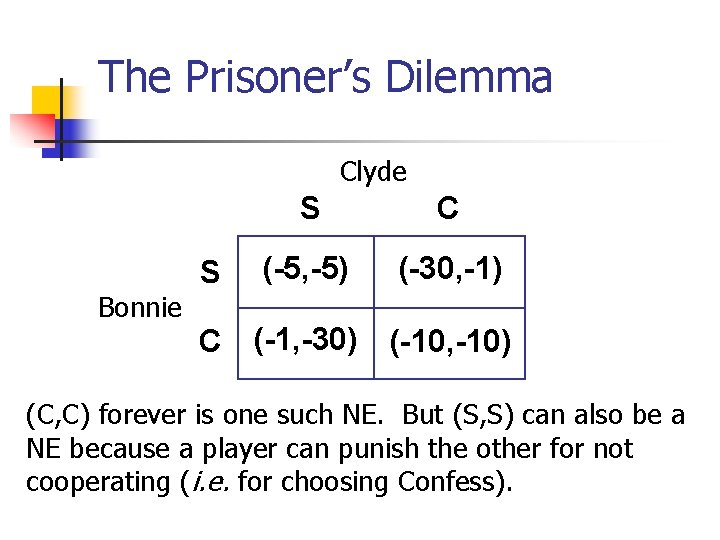The Prisoner’s Dilemma Clyde Bonnie S S C (-5, -5) (-30, -1) C (-1, -30) (-10, -10) (C, C) forever is one such NE. But (S, S) can also be a NE because a player can punish the other for not cooperating (i. e. for choosing Confess).